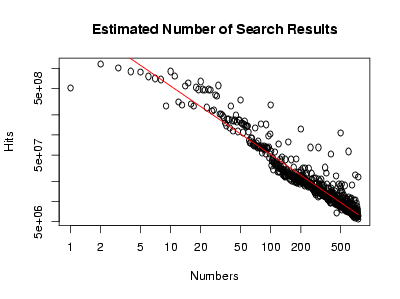# Most numbers are boring, asymptotically speaking.

##### December 10, 2006 personal

Let be the number of Google hits for the integer . Then is about 100 million, and , that is, the number of hits for a number twice as big, is about 40 million, a bit less than half as big. Doubling the input continues to halve the output: is about 20 million (half again!), and is about 8 million, and is about 4 million.

There are about half as many pages talking about numbers that are twice as big. This is an example of a power law, and indeed, a log-log plot of looks linear to my blurry vision:Doing a linear regression in R gives the red line, or in symbols, Rather humorously, this means that . In the end, this is not so surprising: Zipf’s law says that, in a corpus of naturally occuring text, the frequency of a word is inversely proportional to its rank; here, we have a similar phenomenon at work: roughly, the popularity of a number is inversely proportional to its size.

In other words, while the number of integers expressible with fewer than bits grows exponentially in , the number of pages discussing integers expressible with fewer than bits grows linearly in ; being silly, I’d say that this is an asymptotic version of the claim that most large numbers are uninteresting. After all, popular numbers have a lot of fan sites.